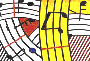Tutorial 18 - Generation of a note sequence by interpolation between two chords

Topics

Random sequencing of interpolated chords

Functions used

interpolation, omloop, nth-random, repeat-n, flat,CHORD and CHORD-SEQ.

Description

This tutorial will again use random generated notes from particular chords to construct a sequence as it is the case in tutorial 17. The difference with the preceding tutorial will be that these chords are the result of an interpolation between two chords carefully choosen. The first chord is in the lower register, whereas the second is in the upper register. The result of the interpolation will be an ascending sequence of chords and so will be the general tendancy of our generated note sequence.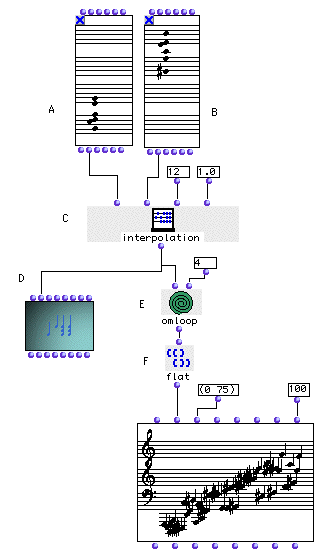Patch structure

A: We have choosen two chords of same density (i.e same number of notes) in CHORD (A) and in CHORD (B) the first is in th elower register and the second in the upper register.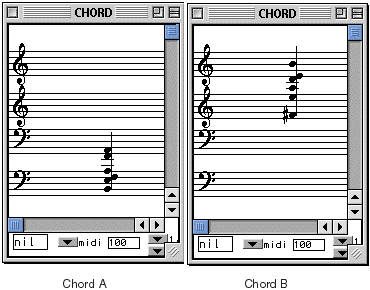C: interpolation (C) will interpolate between these two chords n number of times. In our example above we have choosen to interpolate by 12 times (chord A and B included). The last argument of interpolation is the curve coresponding to the interpolation tendancy. 1 is linear, meaning that we will interpolate step by step having 50% of A in the first 6 chords and 50% of B in the other way.In non-linear interpolation we could move faster toward an extremity or another depending on the desired curve (please consult the OpenMusic Reference Guide for further information abpout this module).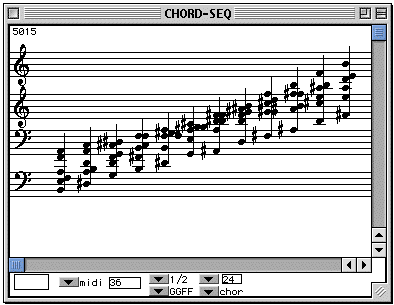D: The CHORD-SEQ (D) shows the resulting interpolation.

E: In order to apply the same process as in tutorial15, (picking randomly n-samples of each chord from the interpolation) we will use omloop (E).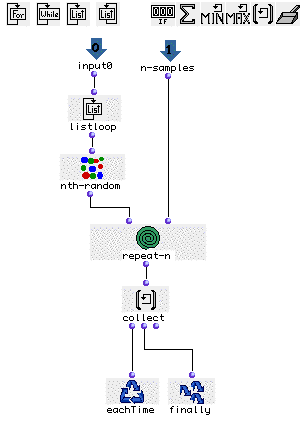In the input0 we will enumerate each chord with listloop. nth-random will randomly choose one note of this chord. Connecting nth-random's output to a repeat-n module will repeat n-times the process for the same chord. Notice that repeat-n's second input is connected to an additional omloop's input that we named 'n-samples' in order to choose the number of times we want to sample each chord from outside omloop's editor window (in our example it is 4 notes per chord, see general patch). All results will be collected by collect and ouput by finally.

F: The output of omloop will be a list of list equivalent to a list of chords. In order to have a sequence of note instead of chords, one must remove the inner level of parenthesis by using the flat function (F). Now we can connect the ouput of flat to the second input of a CHORD-SEQ to play our sequence.